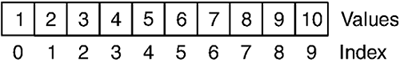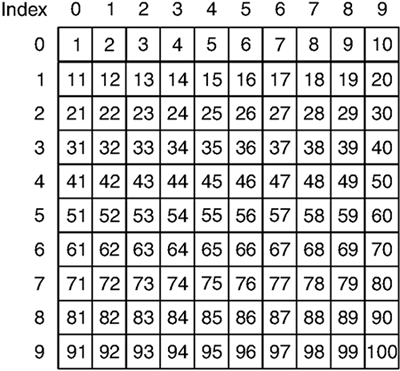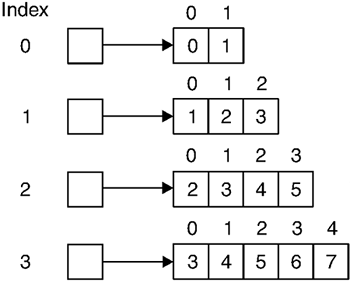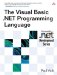# Arrays

 <  Day Day Up  >

An array is a type that contains a set of variables of the same type that are accessed by position rather than by name . The individual variables in the array are called the elements of the array; the type of the variables is called the element type of the array. A variable at a particular position in an array is referenced by number, which is called the index of the element. For example, the following code creates a ten-element array of Integer and fills it with the values 0 through 9.

` Dim a() As Integer Dim i As Integer ReDim a(9) For i = 0 To 9   a(i) = i Next i `

The name of an array type is the element type of the array followed by a set of parentheses ”for example, Integer() . In most cases, the parentheses can be placed on the variable name instead of the type name for emphasis, as in the previous example. The two declaration types are completely equivalent. The parentheses after the element type of an array indicate the number of dimensions in the array, also called the rank of the array. A one-dimensional array is like a list of variables, as illustrated in Figure 4-1. A two-dimensional array is like a matrix, as illustrated in Figure 4-2.

##### Figure 4-1. A One-Dimensional Array##### Figure 4-2. A Two-Dimensional ArrayAn array can have up to 32 dimensions. The rank of an array is represented by commas between the parentheses in the type name. The type Integer() has one dimension, the type Integer(,) has two dimensions, the type Integer(,,) has three dimensions, and so on. The following example creates a two-dimensional array of Integer and fills it with values.

` Dim a(,) As Integer Dim x, y As Integer ReDim a(9, 9) For x = 0 To 9   For y = 0 To 9     a(x, y) = x * y   Next y Next x `

#### ReDim and Erase Statements

Arrays must be created and given a size before they can be used. (This is because they are reference types, a topic covered in Chapter 9, Classes and Structures.) The ReDim statement can be used to allocate a new array with a particular set of dimension sizes.

` Dim a() As Integer Dim b(,) As Double ReDim a(9), b(3,4) `

The length of a dimension in a ReDim statement is specified in terms of its upper bound. So the statement ReDim a(9, 9) creates an array of two dimensions with ten elements (0 through 9) in each dimension. The number of dimensions specified in a ReDim statement must be the same as the number of dimensions of the array type. When an array is dimensioned, each element in the array is initialized to the default value of the array element type. Multiple arrays may be dimensioned at one time, even if they have different element types and dimensions.

It is possible to use ReDim to change the size of the dimensions of an existing array. In that case, the values contained in the array are discarded unless the keyword Preserve is specified following the ReDim keyword. If Preserve is specified and the new array is bigger than the old array, the new elements are initialized to the default value of the array element type. If Preserve is specified and the new array is smaller than the old array, the elements that no longer fit are discarded. The following example will print the numbers 0 through 9 and then ten 0s.

` Dim a() As Integer Dim i As Integer ReDim a(9) For i = 0 To 9   a(i) = i Next i ReDim Preserve a(19) For i = 0 to 19   Console.WriteLine(a(i)) Next i `

## Compatibility

The number of dimensions and/or the element type of the array could be changed as part of a ReDim statement in previous versions. This is no longer allowed because Framework arrays do not have this capability.

The Erase statement clears one or more arrays, resetting them to their original uninitialized state, and is the equivalent of assigning Nothing to the array variable. Because arrays take up space in memory, it is a good practice to erase arrays once they are no longer in use if the variable is going to exist for a long time.

` Dim a(,) As Integer ReDim a(32767, 32767) ' Manipulate the array ... ' Free the memory Erase a ' Do some large amount of work ... `

In a situation where a variable is going to exist for only a short time ”say, a local variable in a small subroutine ”it is not necessary to erase arrays.

## Style

The Erase statement was the only way to clear an array in previous versions. Another way to clear an array in Visual Basic .NET is to set the array variable to Nothing . The two methods are exactly equivalent, and there is no advantage to one over the other.

#### Array Initializers

When you are declaring a variable of an array type, the variable can be initialized at the same time with an array of a particular size or with particular values. An array is initialized to a particular size at declaration time by providing the sizes of each dimension in the parentheses.

` Dim a(,) As Integer Dim b(3,3) As Integer a = b ReDim b(9,9) `

In the example, the variable a is not initialized to anything ”it starts out empty (i.e., initialized to the value Nothing ). However, the variable b is initialized to a two-dimensional array with a length of 4 in both dimensions. As the rest of the example shows, initializing an array variable with an array of a particular length does not fix the size of the array in the variable. It is possible to redimension the variable later on, and it will take on the new size.

The actual values of an array can be specified by following the declaration with an equals sign and the values listed between curly brackets. When an array variable is initialized with a list of values, an explicit array size cannot be supplied ”the size is derived implicitly from the size of the value list. The following example shows several arrays initialized to various sets of values.

` Dim a() As Integer = { 1, 2, 3, 4, 5 } Dim b() As String = { "one", "two", "three", "four", "five" } Dim c(,) As Integer = { { 1, 2, 3 }, { 4, 5, 6 } } `

As the preceding example shows, multidimensional arrays can be initialized by nesting the brackets. Each level of bracketing represents one dimension of the array, with the outermost level of bracketing representing the leftmost dimension and the innermost level of bracketing representing the rightmost dimension. In the example, the variable c is initialized with a two-by-three array. The number of elements at any particular level of the multidimensional array initializer must be the same length.

#### Arrays of Arrays

The element type of an array can be any type, including another array. An array whose element type is an array is indicated by adding another set of parentheses to the end of the type name. For example, the type name of a one-dimensional array of one-dimensional array of Integer is Integer()() . The important thing to keep in mind is that the element type of Integer()() is Integer() ”thus, you have to first dimension each element of the outer array before you can assign an Integer value to an element of an inner array. In contrast, the element type of Integer(,) is Integer , which means you can always assign a value to an element in the array. Compare this example to the one in the previous section.

` Dim a()() As Integer Dim x, y As Integer ReDim a(3) For x = 0 To 3   ReDim a(x)(x + 1)   For y = 0 To x + 1     a(x)(y) = x + y   Next y Next x `

In the example, it is necessary to dimension each inner array that is an element of the outer array. Because arrays of arrays are usually asymmetrical , they are also known as jagged arrays . The array produced by the preceding example looks something like Figure 4-3.

##### Figure 4-3. A Jagged ArrayArrays of arrays are useful when an array has more than one dimension but the dimensions are not symmetrical. For example, imagine a soccer league with 12 teams that can be made up of 11 to 18 players. The names of all the members could be stored in a two-dimensional array of String (i.e., String(,) ) whose dimensions are 12 by 18, but this would waste extra space if teams had fewer than 18 players. Instead, a one-dimensional array of the one-dimensional array of String (i.e., String()() ) could be used. The dimension of the outer array would be 12, but the dimension of the inner array could be anywhere from 11 to 18. For example:

` Dim Teams(12)() As Integer Dim Team As Integer For Team = 0 To 9   Dim TeamSize As Integer   Dim Member As Integer   Console.WriteLine("How many players are on team " & Team & "?")   TeamSize = CInt(Console.ReadLine())   ReDim Teams(Team)(TeamSize - 1)   For Member = 0 To TeamSize  1     Console.WriteLine("What is player " & Member & "'s name?")     Teams(Team)(Member) = Console.ReadLine()   Next Member Next Team `

Arrays of arrays may be nested to any level and may be of any dimension. For example, the type Integer()(,)(,,) is an array of two-dimensional arrays of three-dimensional arrays of Integer .

Accessing the elements of a one-dimensional array is faster than accessing the elements of a multidimensional array on most versions of the .NET Framework. As a result, if performance is a consideration, it may be faster to use jagged arrays than regular multidimensional arrays.

 <  Day Day Up  >The Visual Basic .NET Programming Language
ISBN: 0321169514
EAN: 2147483647
Year: 2004
Pages: 173
Authors: Paul Vick

Similar book on Amazon